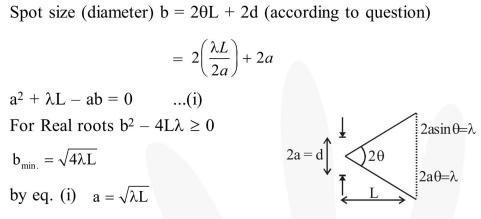Deepak Scored 45->99%ile with Bounce Back Crack Course. You can do it too!

# The box of a pin hole camera, of length

Question:

The box of a pin hole camera, of length $\mathrm{L}$, has a hole of radius a. It is assumed that when the hole is illuminated by a parallel beam of light of wavelength $\lambda$ the spread of the spot (obtained on the opposite wall of the camera) is the sum of its geometrical spread and the spread due to diffraction. The spot would then have its minimum size $\left(\right.$ say $b_{\min }$ ) when :-

1. $a=\frac{\lambda^{2}}{L}$ and $b_{\min }=\sqrt{4 \lambda L}$

2. $a=\frac{\lambda^{2}}{L}$ and $b_{\min }=\left(\frac{2 \lambda^{2}}{L}\right)$

3. $\mathrm{a}=\sqrt{\lambda \mathrm{L}}$ and $\mathrm{b}_{\min }=\left(\frac{2 \lambda^{2}}{\mathrm{~L}}\right)$

4. $a=\sqrt{\lambda L}$ and $b_{\min }=\sqrt{4 \lambda L}$

Correct Option: , 4

Solution: